# FEM model¶

## Finite Element Modelling (FEM) of conical indentation¶

The present model is a simulation of the conical nanoindentation process, using the FEM software ABAQUS.

The Matlab function used to generate a Python script for ABAQUS is : python_abaqus

The model is axisymmetric with a geometry dependent mesh and restricted boundaries conditions.

### Geometry of the (multilayer) sample¶

Each layer of the sample is characterized by its thickness ($$t_\text{i}$$).

The thickness of the substrate ($$t_\text{sub}$$) is set as 2 times the highest thin film thickness.

The width ($$w$$) of the sample is calculated in function of the substrate thickness or the indenter tip defect.

No delamination is allowed between thin films or between thin film and substrate.

Note

Dimensions are in nm.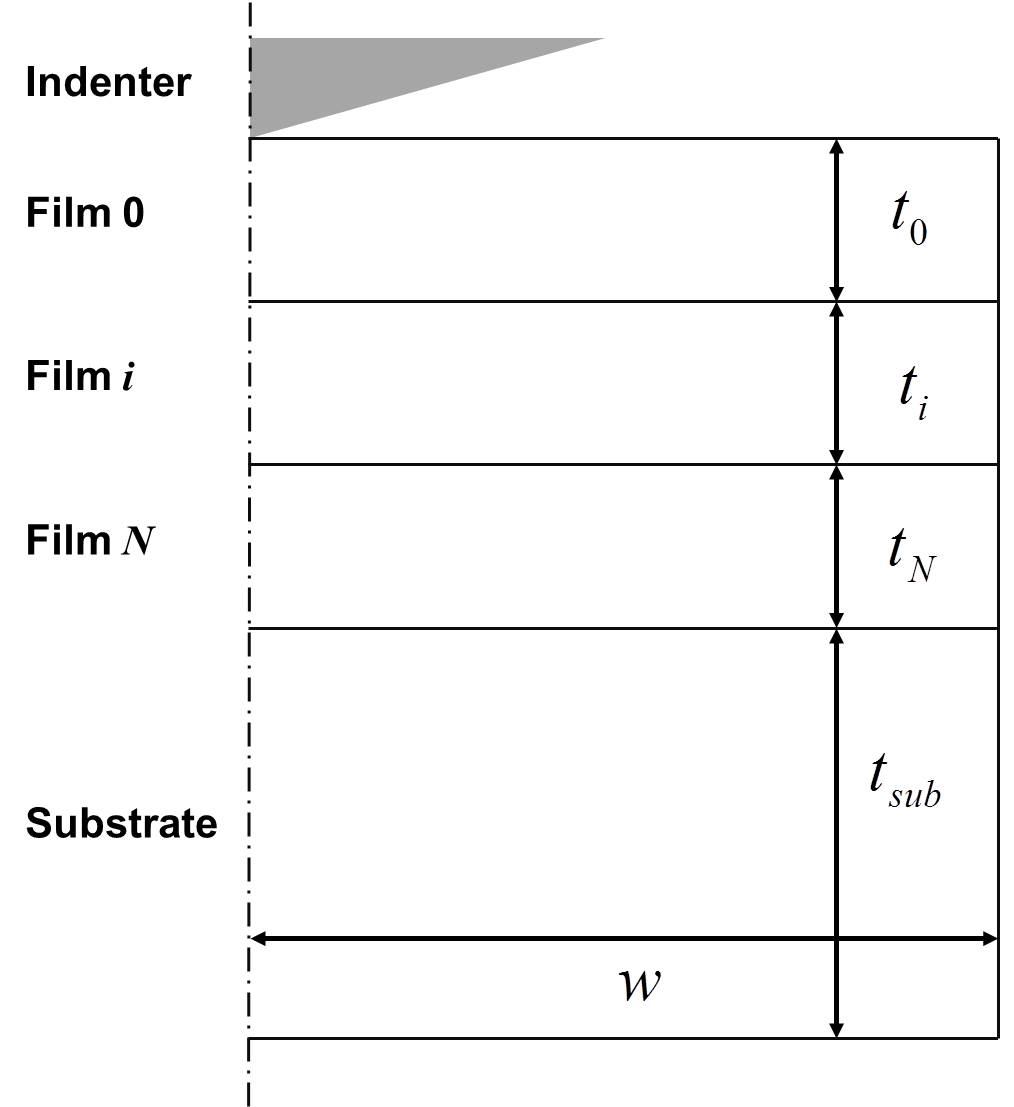Figure 29 Geometry of the sample in the FEM model.

### Geometry of the indenter¶

The indenter is defined as a rigid cono-spherical indenter. A spherical part is defined at the apex of the conical indenter (see Figure 30).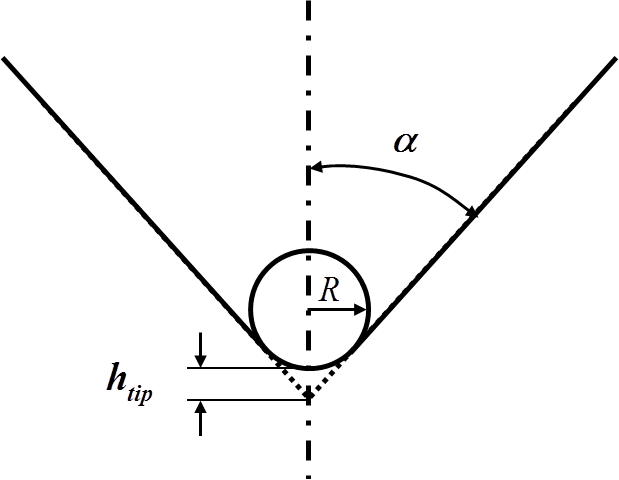Figure 30 Scheme of a cono-spherical indenter.

The radius $$R$$ of the spherical part is calculated from the tip defect $$h_\text{tip}$$ and the cone angle $$\alpha$$, using the following equation. For Berkovich, Vickers and Cube-Corner indenters, the equivalent cone angle is used to set the cone angle.

(97)$R = \frac{h_\text{tip}}{\frac{1}{sin(\alpha)} - 1}$

In case, a perfect conical indenter is set in the GUI ($$h_\text{tip} = 0\text{nm}$$), the radius is calculated using a tip defect of 0.1nm by default, which gives a radius of $$R = 1.6\text{nm}$$.

Defining a spherical tip avoids the geometrical singularity at the apex of the perfect conical indenter, which would imply an infinite stress at the contact interface.

### Mesh¶

The multilayer sample is divided by default into solid elements with eight nodes and axisymmetric deformation element CAX8R is adopted.

It is possible to divide the sample into solid elements with four nodes and with axisymmetric deformation element CAX4R, by changing the value of the variable “linear_elements” in the Matlab function python4abaqus from 0 (quadratic elements) to 1 (linear elements).

Note

• CAX4R: A 4-node bilinear axisymmetric quadrilateral, reduced integration, hourglass control.
• CAX3: A 3-node linear axisymmetric triangle.
• CAX6M: A 6-node modified quadratic axisymmetric triangle.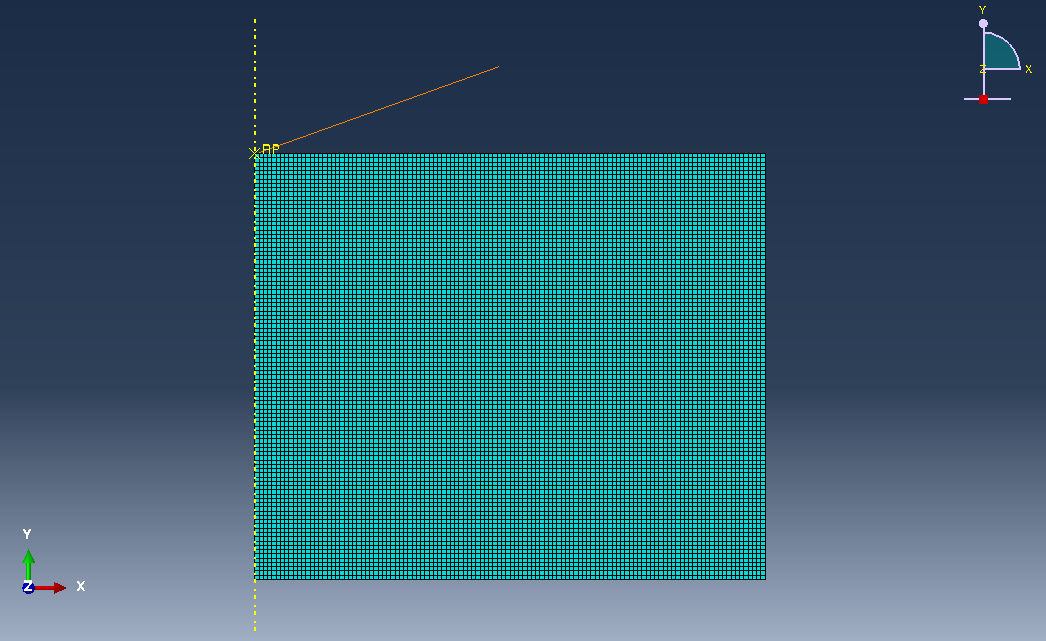Figure 31 Screenshot in Abaqus of the mesh example used in the FE model.

### Material properties¶

For each layers of the multilayer sample, the material properties (Young’s modulus and Poisson’s ratio) are defined using the inputs given by the user from the GUI. Material properties are considered by default to be isotropic. The density is set by default to 1.0.

Note

Young’s moduli are in GPa.

### Contact definition¶

The contact is defined by default frictionless for the tangential behavior and hard for the normal behavior.

The external surface of the indenter is defined as the “master” region and the top surface of the (multilayer) sample is defined as the “slave” region.

Note

Usually, the effect of friction may be neglected when indenter tips with half-angle larger than 60◦ are used (e.g.: Berkovich, Vickers) , , , .

### Boundaries conditions¶

Nodes are constrained along the rotation axis from moving in the radial direction ($$x$$). The nodes on the bottom surface of the sample are constrained along the radial axis from moving in the radial ($$x$$) and vertical ($$z$$) directions (see Figure 32 and Figure 33).

Indentation process is simulated by imposing a vertical displacement to the rigid indenter along the ($$z$$) axis (see Figure 32 and Figure 33). A value of 200nm for the indentation depth is set by default.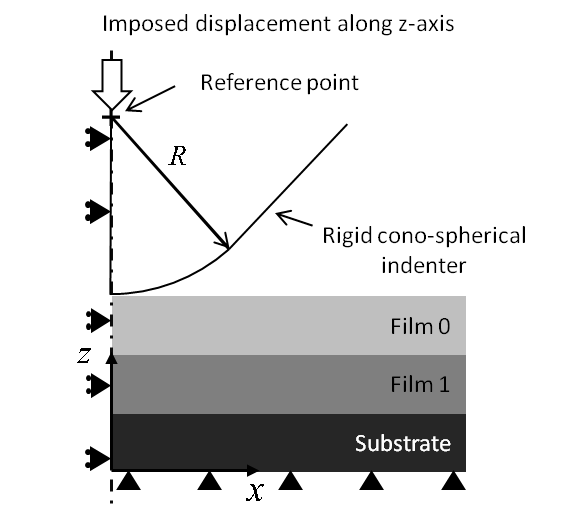Figure 32 Schematic of boundaries conditions used in the FE model.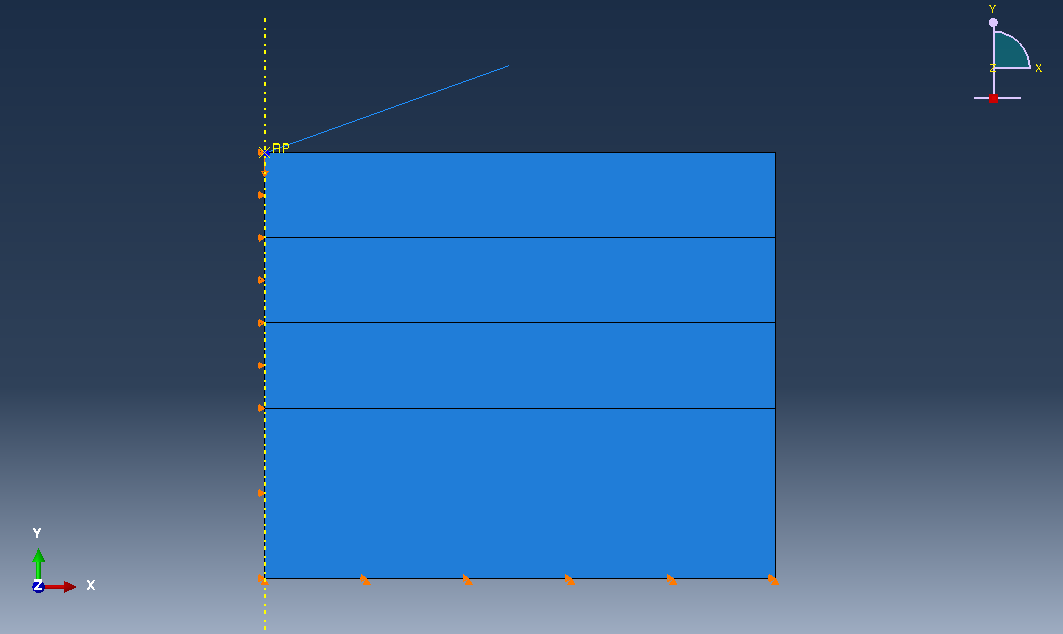Figure 33 Screenshot of the FE model with BCs in Abaqus.

Warning

Indentation displacement is given in nanometers and is negative.

## Generation of the Python script for ABAQUS¶

After material properties are configured (Young’s moduli and Poisson’s ratios) and the model geometry is given (thickness for each thin films), a Python script for ABAQUS can be generated by pressing the ‘FEM’ button.

The python script is saved in the folder where your nanoindentation results are stored.

To generate the FEM model in ABAQUS, apply the following procedure:

• start ABAQUS
• select the folder containing input files : ‘File’ ==> ‘Set Work Directory…’
• select and run the Python file containing the FEM model (*.py) : File’ ==> ‘Run Script’

Note

Dimensions are in nm and Young’s moduli are in GPa, implying that load is in nN.

## Results of the FEM simulation¶

The following pictures were obtained for a multilayer Au/Ti/SiO2/Si.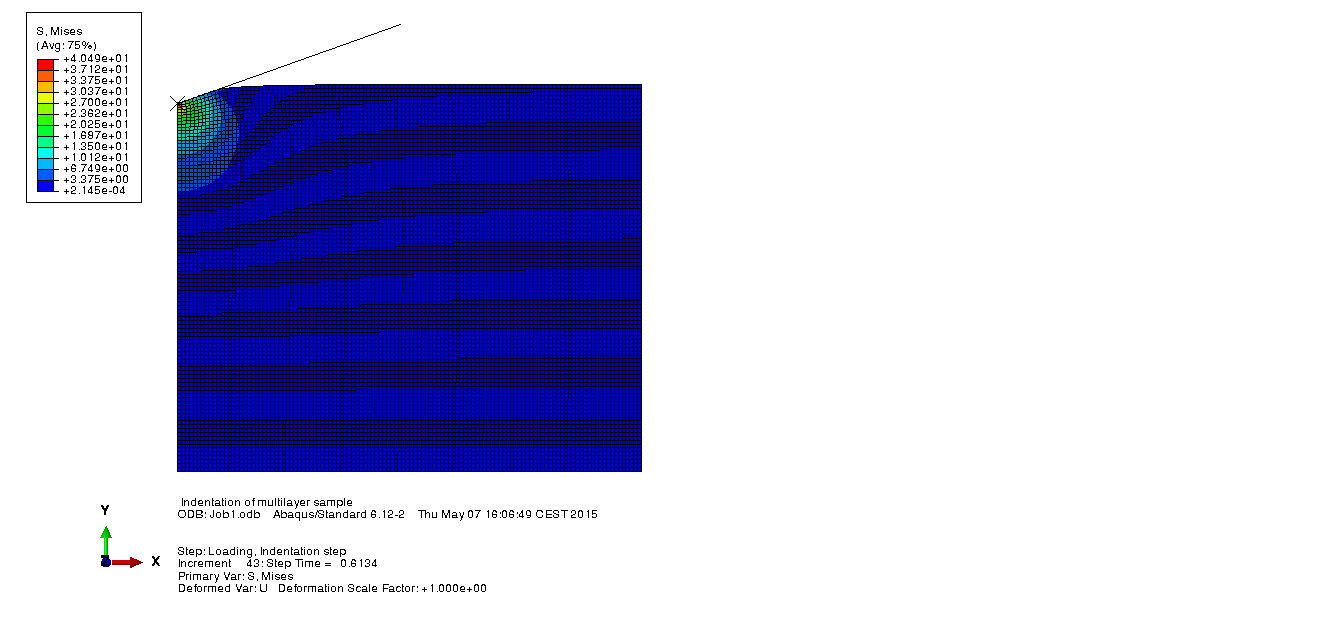Figure 34 Screenshot of the Von Mises stress distribution at maximum load.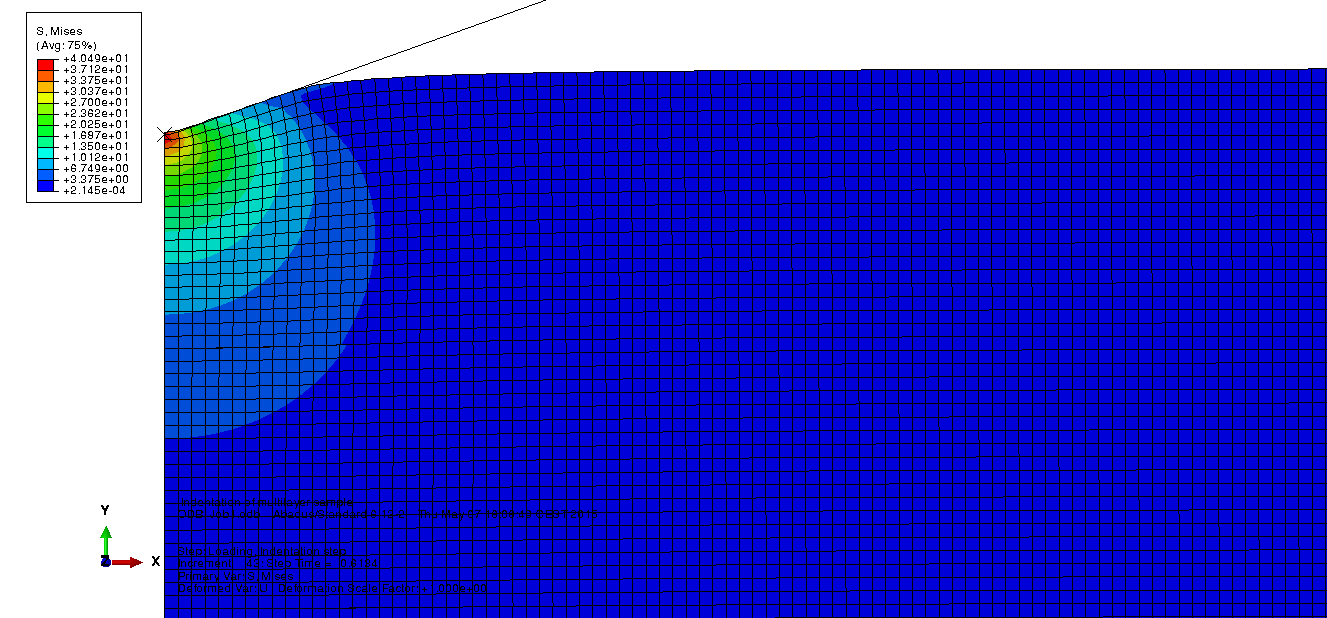Figure 35 Screenshot (with a zoom in on the contact area) of the Von Mises stress distribution at maximum load.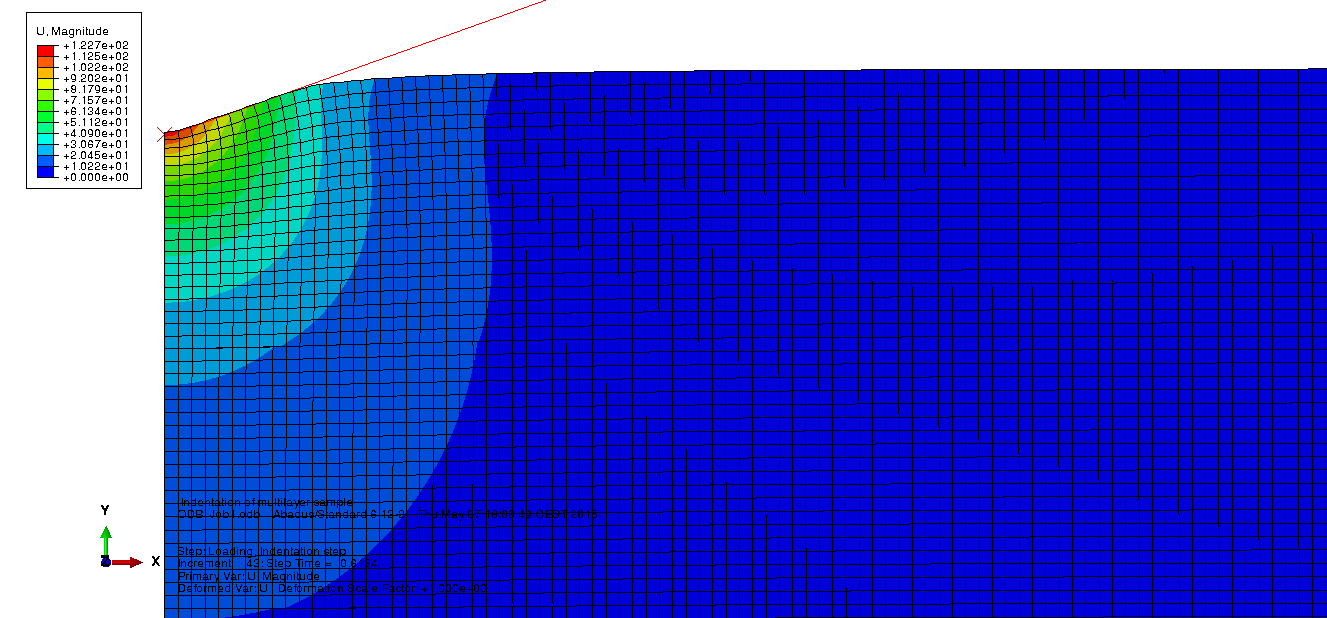Figure 36 Screenshot (with a zoom in on the contact area) of the magnitude of the displacement at maximum load.